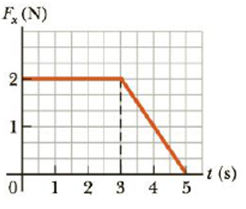Chapter 6, Problem 15P

Chapter
Section
Textbook Problem

The force shown in the force vs. time diagram in Figure P6.15 acts on a 1.5-kg object. Find (a) the impulse of the force, (b) the final velocity of the object if it is initially at rest, and (c) the final velocity of the object if it is initially moving along the x-axis with a velocity of −2.0 m/s.Figure P6.15

(a)

To determine
The Impulse of the force.

Explanation

Given info The Mass of the object is m=1.5kg

From the figure,

The Height of the rectangle is 3.0, width of the rectangle is 2.0N,base of the triangle is 2.0s and altitude of the triangle is 2.0N.

The expression for impulseis

I=Arec+Atri

• Arec is the area of the rectangle,
• Atri is the area of the triangle,
• I is the impulse,

Rewrite the above expression.

I=(hw)rec+12(b+a)tri                                                   (1)

• h is the height,
• w is the width
• b is the base,
• a is the altitude,

Substitute 3

(b)

To determine
The final velocity of the object initially at rest.

(c)

To determine
Final velocity of the object initially moving along the x axis.

Still sussing out bartleby?

Check out a sample textbook solution.

See a sample solution

The Solution to Your Study Problems

Bartleby provides explanations to thousands of textbook problems written by our experts, many with advanced degrees!

Get Started

Find more solutions based on key concepts